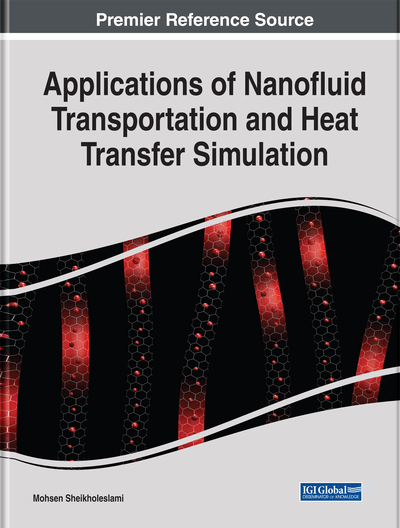# Discharging of Nano-Enhanced PCM via Finite Element Method

DOI: 10.4018/978-1-5225-7595-5.ch004

## Abstract

Latent heat thermal energy storage systems (LHTESS), which work based on energy storage and retrieval during solid-liquid phase change, is used to establish balance between energy supply and demand. LHTESS stores and retrieves thermal energy during solid-liquid phase change, while in SHTESS phase change doesn't occur during the energy storage and retrieval process. LHTESS has a lot of advantages in comparison to SHTESS. The most important one is storing a large amount of energy during phase change process, which makes the energy storage density in LHTESS much higher than SHTESS. Because of this property, LHTESS have a wide application in different cases, such as solar air dryer, HVAC systems, electronic chip cooling, and engine heat recovery. The main restriction for these systems is thermal conductivity weakness of common PCMs. In this chapter, the method of adding nanoparticles to pure PCM and making nano-enhanced phase change material (NEPCM) and using fin with suitable array are presented to accelerate solidification process. The numerical approach which is used in this chapter is standard Galerkin finite element method.
Chapter Preview
Top

## 1. Discharging Process Expedition Of Nepcm In Y-Shaped Fin-Assisted Latent Heat Thermal Energy Storage System

### 1.1. Problem Definition and Mathematical Model

#### 1.1.1. Problem Definition

The main geometry of present study is a Fin-assisted LHTESS, which HTF exists in the inner tube, and in the space between inner and outer tube, is filled by PCM. Y-shaped fin is connected to the outer side of HTF tube to improve heat transfer from HTF to PCM (Figure 1) (Lohrasbi, Sheikholeslami, & Ganji, 2016). The two-dimensional solution domain is presented in Figure 2. The angle between fin branches and axes is assumed to be, Fin length and thickness assumed to be equal toandrespectively. The constant temperature boundary condition equal to 240K is applied to inner tube and the initial temperature of liquid PCM is assumed equal to 278K. The PCM, Nanoparticles and fin properties are listed in Table 1.

Table 1.
The physical properties of water as PCM, Copper as nanoparticles and aluminum fin

The transient Governing equations of conduction-dominated solidification process are presented as below:

(1)(2)

When pure PCM is applied in LHTESS, pure PCM properties are used in governing equations but when copper nanoparticles are dispersed in PCM, the NEPCM properties should be obtained by using the following equations and be applied in governing equations (Sheikholeslami, 2014):

(3)(4)(5)

In present study, thermal conductivity of NEPCM is evaluated by the following equation based on Maxwell (Sheikholeslami, 2014) work.

(6) whereandare nanoparticle volume fraction, nanoparticle and base fluid thermal conductivity respectively.

Total energy density released during discharging process, is calculated from the following equation:

(7)

#### 1.1.2. Numerical Method

Standard Galerkin Finite Element Method with cubic interpolation over triangles is implemented to solve the present phase change problem. Nodal values are placed on corners and sides of the grid cells. The Galerkin equations are formed by symbolic analysis, which substitutes definitions, segregates dependencies on variables, applies integration by parts, integrates over cells, and ultimately differentiates the resulting system with respect to system variables to form the coupling matrix. Equations are solved simultaneously by an iterative method. For nonlinear systems Newton-Raphson iteration process with back-tracking is used. For time dependent problems, such as solidification problem, an implicit Backward Difference Method for integration in time is used. Variables are approximated by quadratic polynomials in time, and the time step is controlled to keep the cubic term smaller than the required value of error. The residual Galerkin integral over a patch of cells surrounding each mesh node is minimized by the Finite Element Equations. Then the residuals in each cell independently are analyzed as a measure of compliance, and subdivide each cell in which the required error tolerance is exceeded. Any cell thus split can be re-merged whenever the cell error drops to of the splitting tolerance. Adaptive grid refinement is used to simulate solidification process in present work. When the initial mesh generation is performed, code estimates the error and refines mesh in order to reach to the desired accuracy. In unsteady problems, this procedure also has to be applied to the initial values of the variables in order to refine the mesh where rapid change in variables occur (Figure 3). Comparison between the present code based on Galerkin Finite Element Method and experimental results obtained by Ismaeil et al. (2001) indicates good agreement, which validates the present code (see Figure 4). Moreover it proves that ignoring natural convection in numerical simulation of solidification phenomenon leads to results close to reality.

## Complete Chapter List

Search this Book:
Reset